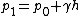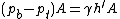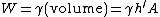Archimedes' principle

Also found in: Dictionary, Thesaurus, Medical.

Archimedes' principle,

principle that states that a body immersed in a fluid is buoyed up by a force equal to the weight of the displaced fluid. The principle applies to both floating and submerged bodies and to all fluids, i.e., liquids and gases. It explains not only the buoyancy of ships and other vessels in water but also the rise of a balloon in the air and the apparent loss of weight of objects underwater. In determining whether a given body will float in a given fluid, both weight and volume must be considered; that is, the relative densitydensity,
ratio of the mass of a substance to its volume, expressed, for example, in units of grams per cubic centimeter or pounds per cubic foot. The density of a pure substance varies little from sample to sample and is often considered a characteristic property of the
, or weight per unit of volume, of the body compared to the fluid determines the buoyant force. If the body is less dense than the fluid, it will float or, in the case of a balloon, it will rise. If the body is denser than the fluid, it will sink. Relative density also determines the proportion of a floating body that will be submerged in a fluid. If the body is two thirds as dense as the fluid, then two thirds of its volume will be submerged, displacing in the process a volume of fluid whose weight is equal to the entire weight of the body. In the case of a submerged body, the apparent weight of the body is equal to its weight in air less the weight of an equal volume of fluid. The fluid most often encountered in applications of Archimedes' principle is water, and the specific gravityspecific gravity,
ratio of the weight of a given volume of a substance to the weight of an equal volume of some reference substance, or, equivalently, the ratio of the masses of equal volumes of the two substances.
of a substance is a convenient measure of its relative density compared to water. In calculating the buoyant force on a body, however, one must also take into account the shape and position of the body. A steel rowboat placed on end into the water will sink because the density of steel is much greater than that of water. However, in its normal, keel-down position, the effective volume of the boat includes all the air inside it, so that its average density is then less than that of water, and as a result it will float.

Archimedes' principle

The principle that the net fluid force on a body submerged (or floating) in a stationary fluid is an upward force equal to the weight of the fluid displaced by the body. This concept, perhaps the oldest stated principle in fluid mechanics, was first put forth by Archimedes in the third century B.C.

In a static fluid, the weight of the fluid causes an increase in pressure with depth. Thus, at the surface of the fluid, the pressure is atmospheric pressure (p0 = 14.7 lb/in.2 = 101 kilonewtons/m2), while at a depth h the pressure has a larger value of p1, given by

(1)Eq. (1), where γ is the specific weight of the fluid (weight/volume). The difference in pressure force between the bottom and the top of a water column is therefore given by Eq. (2), where h and A
(2)are the height and area of the column, and pb and pt are the pressures at the bottom and top of the column. This difference is precisely equal to the weight W
(3)of the water within the column, given by Eq. (3). If the water column were replaced with a solid object, the pressure forces on the object would be the same as on the original water column. That is, the net hydrostatic pressure force on the object, termed the buoyant force, would be equal to the weight of the water displaced (which is the statement of Archimedes' principle). The same concept holds for a body of arbitrary shape, which can be thought of a consisting of many small vertical columns fastened together. Archimedes' principle is valid for submerged or floating bodies in liquids or gases. See Buoyancy, Specific gravity

McGraw-Hill Concise Encyclopedia of Physics. © 2002 by The McGraw-Hill Companies, Inc.

Archimedes' principle

a law of physics stating that the apparent upward force (buoyancy) of a body immersed in a fluid is equal to the weight of the displaced fluid
Collins Discovery Encyclopedia, 1st edition © HarperCollins Publishers 2005
References in periodicals archive ?
The same tendency was found in the correlation between stomach volume and stomach content, with the Archimedes' principle method being higher than the Jobling's method ([R.sup.2] values of 0.95 and 0.68, respectively; Fig.
The behaviour of the curves created by plotting the points (X, Y) of the various relationships is as follows: with the Jobling's method, the intersection of the curve with the Y-axis (0.0499, 4.3092 and 3.7867 in Figures 1a-1c, respectively) is higher in each case than with the Archimedes' principle method (0.0007, 1.4467 and 1.6138 in Figures 1a-1c, respectively).
According to the present experiment, the Archimedes' principle method is more effective and provides greater data precision in the measurement of real maximum stomach volume in fish, based on higher [R.sup.2] (means best curve adjustment) compared to results obtained by Jobling method.
According to the relationship between stomach volume and stomach content, the Archimedes' principle method presented a higher coefficient of determination than the Jobling's method; this could be because the first method considers the real maximum volume of the stomach, while the second considers the maximum forced expanded stomach volume.
The intent of this research was to find out if it is possible to get sixth graders--who have not yet been formally taught the concepts of mass, density, pressure, volume and buoyancy--to understand (and, perhaps, arrive at) Archimedes' principle. The entire lesson was therefore presented without using any of these terms.
Helping students develop an understanding of Archimedes' principle II.
The method was validated by comparing the volume of stones measured by Archimedes' principle to the corresponding values estimated with PHM.
Galileo was clearly keeping the scaling theory a secret for the eventuality of a situation like this one, and his new view of Archimedes' principle was completely beyond the abilities of his opponents to answer.
This important discovery later became known as Archimedes' Principle.
Visualising a space where people could learn about Archimedes' principles in a 'hands on' way became a dream he realised in 2014.

Site: Follow: Share:
Open / Close